Example Questions

1 2 7 8 9 10 11 12 13 15 Next →

Example Question #4 : How To Find Range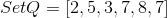Quantity A: The range of Set Q.

Quantity B: The median of Set Q.

Quantity A is greater.

Quantity B is greater.

The two quantities are equal.

The relationship cannot be determined.

The two quantities are equal.

Explanation:

The first step of this problem should be to reorder Set Q into numerical order: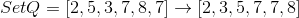The range of a set is the largest value minus the lowest value, so for this set, the range is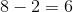The median of a set depends on whether or not there is an even or odd amount of numbers in the set. For a set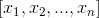withvalues:

Odd: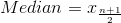Even: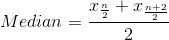Since there is an even number of numbers in this set, the median is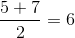The two quantities are equal.

1 2 7 8 9 10 11 12 13 15 Next →

Tired of practice problems?

Try live online GRE prep today.